Скачать презентацию Replacement Analysis and Economic Life Should we replace

21082bdffc9f46216c776d1403eb8894.ppt

• Количество слайдов: 26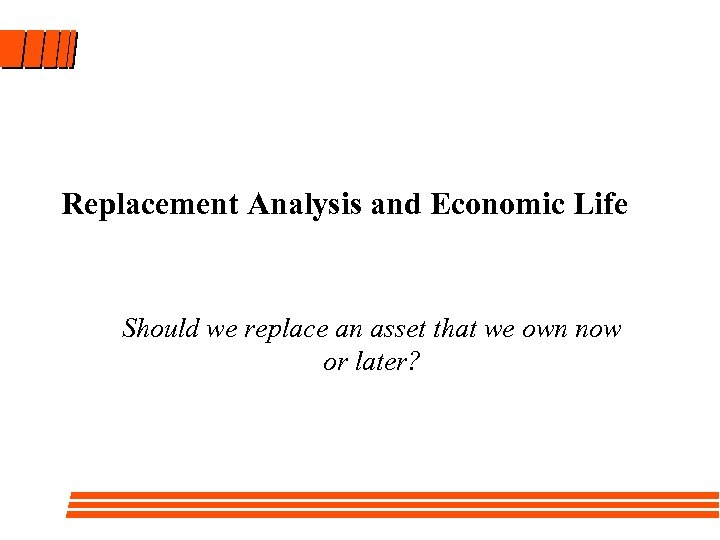Replacement Analysis and Economic Life Should we replace an asset that we own now or later?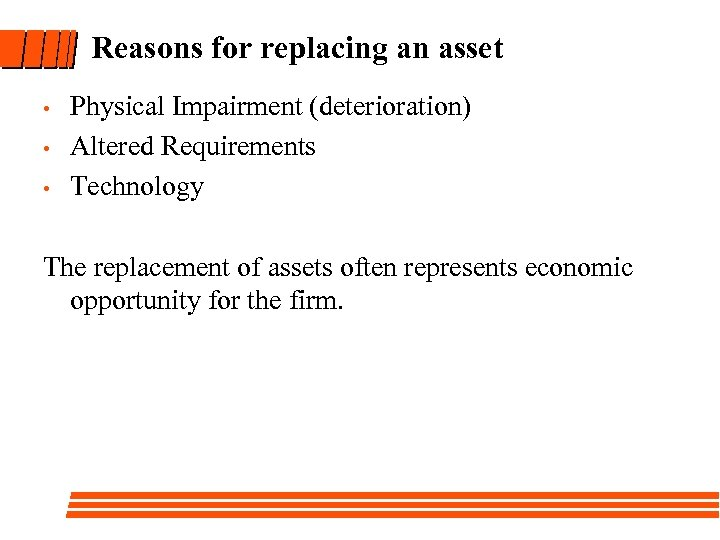Reasons for replacing an asset • • • Physical Impairment (deterioration) Altered Requirements Technology The replacement of assets often represents economic opportunity for the firm.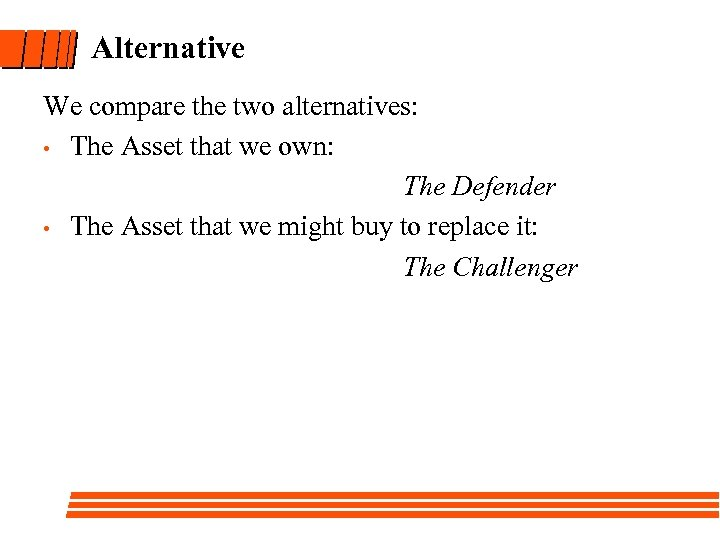Alternative We compare the two alternatives: • The Asset that we own: The Defender • The Asset that we might buy to replace it: The Challenger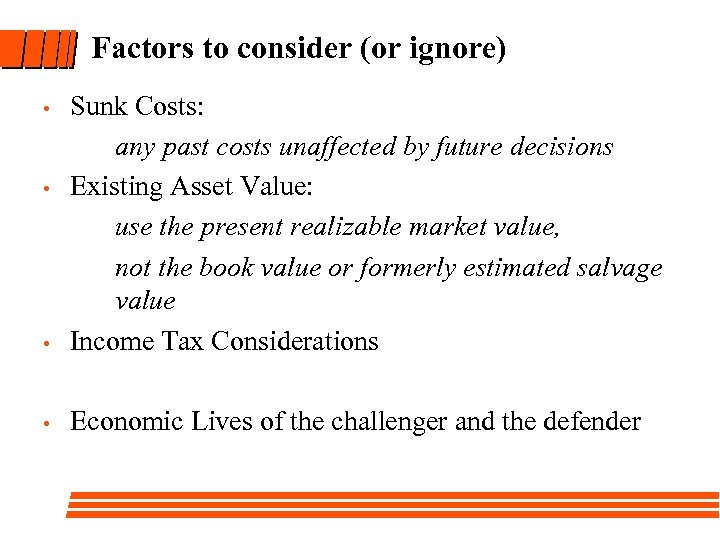Factors to consider (or ignore) Sunk Costs: any past costs unaffected by future decisions • Existing Asset Value: use the present realizable market value, not the book value or formerly estimated salvage value • Income Tax Considerations • Economic Lives of the challenger and the defender •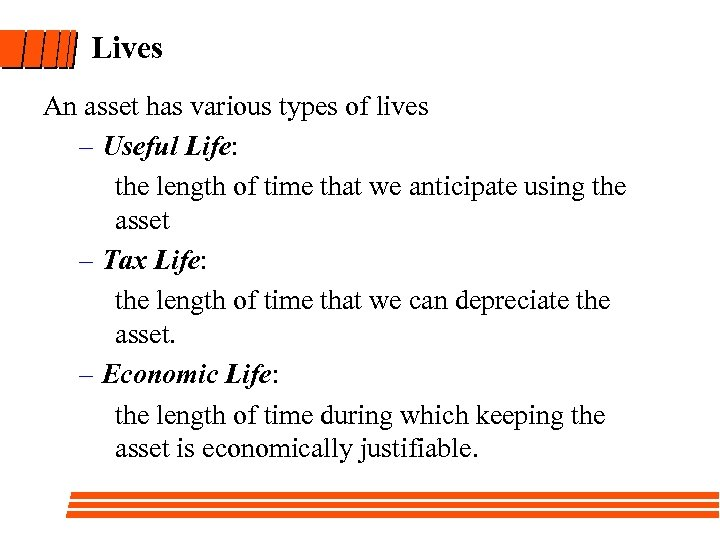Lives An asset has various types of lives – Useful Life: the length of time that we anticipate using the asset – Tax Life: the length of time that we can depreciate the asset. – Economic Life: the length of time during which keeping the asset is economically justifiable.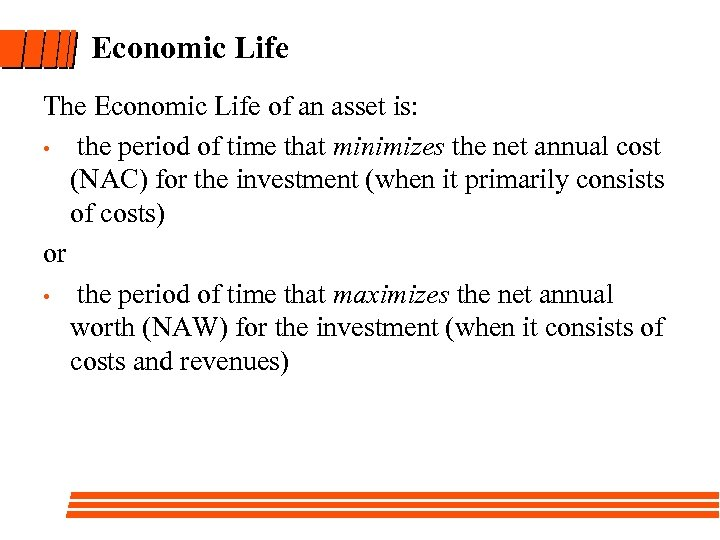Economic Life The Economic Life of an asset is: • the period of time that minimizes the net annual cost (NAC) for the investment (when it primarily consists of costs) or • the period of time that maximizes the net annual worth (NAW) for the investment (when it consists of costs and revenues)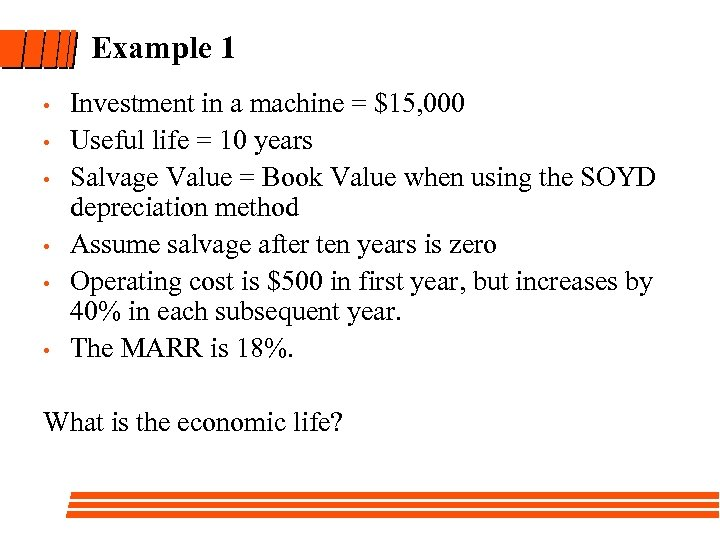Example 1 • • • Investment in a machine = \$15, 000 Useful life = 10 years Salvage Value = Book Value when using the SOYD depreciation method Assume salvage after ten years is zero Operating cost is \$500 in first year, but increases by 40% in each subsequent year. The MARR is 18%. What is the economic life?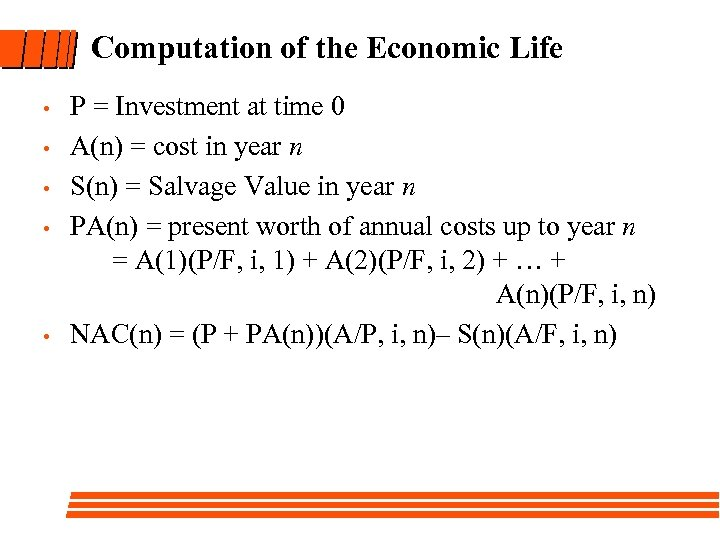Computation of the Economic Life • • • P = Investment at time 0 A(n) = cost in year n S(n) = Salvage Value in year n PA(n) = present worth of annual costs up to year n = A(1)(P/F, i, 1) + A(2)(P/F, i, 2) + … + A(n)(P/F, i, n) NAC(n) = (P + PA(n))(A/P, i, n)– S(n)(A/F, i, n)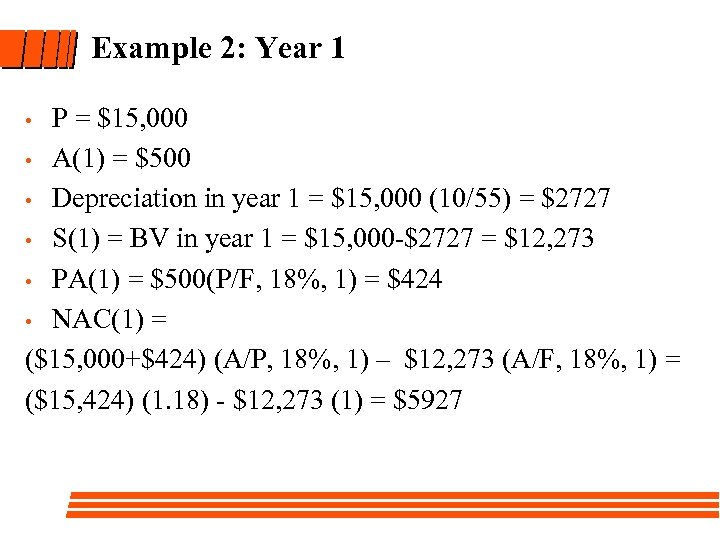Example 2: Year 1 P = \$15, 000 • A(1) = \$500 • Depreciation in year 1 = \$15, 000 (10/55) = \$2727 • S(1) = BV in year 1 = \$15, 000 -\$2727 = \$12, 273 • PA(1) = \$500(P/F, 18%, 1) = \$424 • NAC(1) = (\$15, 000+\$424) (A/P, 18%, 1) – \$12, 273 (A/F, 18%, 1) = (\$15, 424) (1. 18) - \$12, 273 (1) = \$5927 •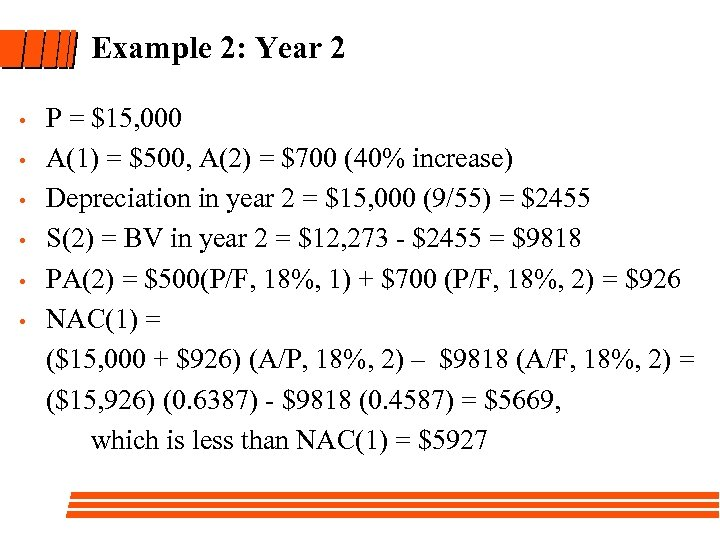Example 2: Year 2 • • • P = \$15, 000 A(1) = \$500, A(2) = \$700 (40% increase) Depreciation in year 2 = \$15, 000 (9/55) = \$2455 S(2) = BV in year 2 = \$12, 273 - \$2455 = \$9818 PA(2) = \$500(P/F, 18%, 1) + \$700 (P/F, 18%, 2) = \$926 NAC(1) = (\$15, 000 + \$926) (A/P, 18%, 2) – \$9818 (A/F, 18%, 2) = (\$15, 926) (0. 6387) - \$9818 (0. 4587) = \$5669, which is less than NAC(1) = \$5927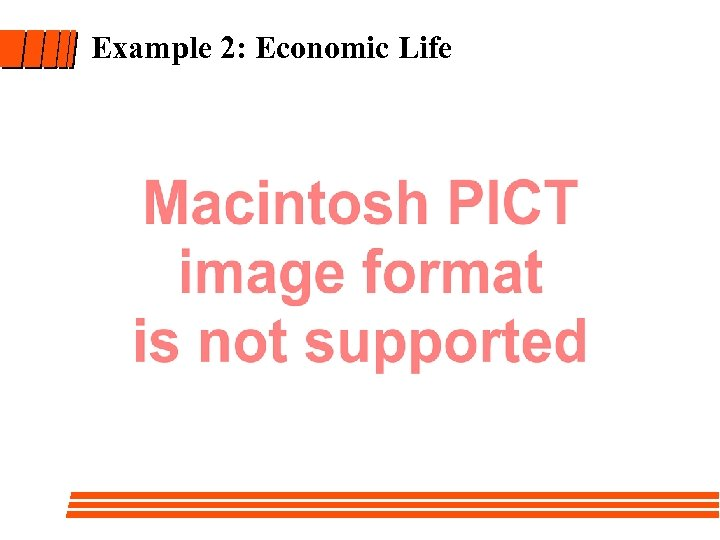Example 2: Economic Life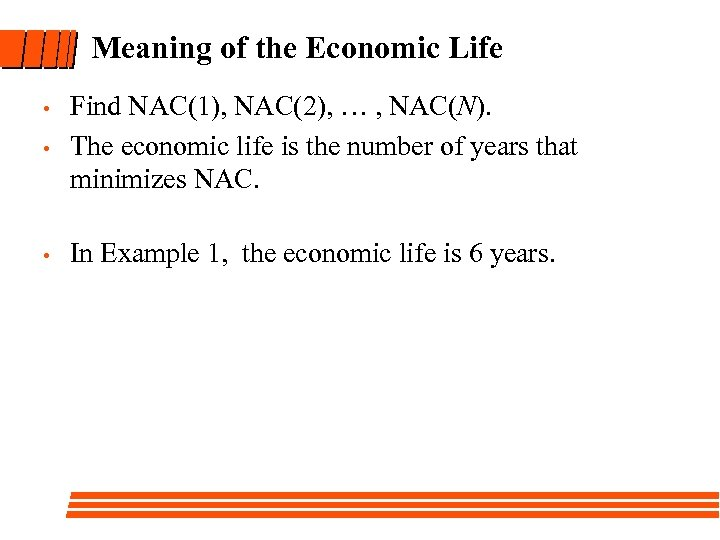Meaning of the Economic Life • Find NAC(1), NAC(2), … , NAC(N). The economic life is the number of years that minimizes NAC. • In Example 1, the economic life is 6 years. •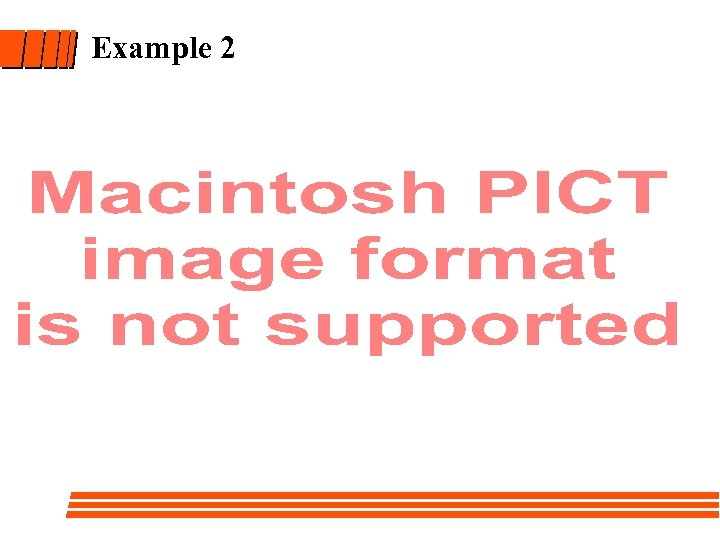Example 2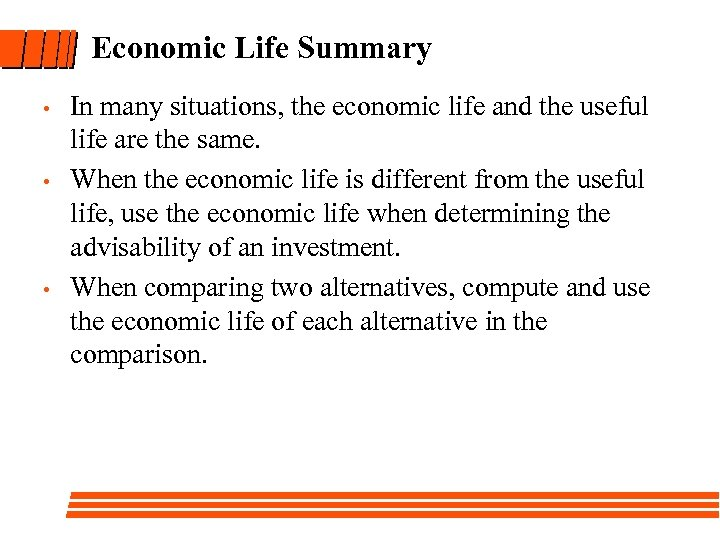Economic Life Summary • • • In many situations, the economic life and the useful life are the same. When the economic life is different from the useful life, use the economic life when determining the advisability of an investment. When comparing two alternatives, compute and use the economic life of each alternative in the comparison.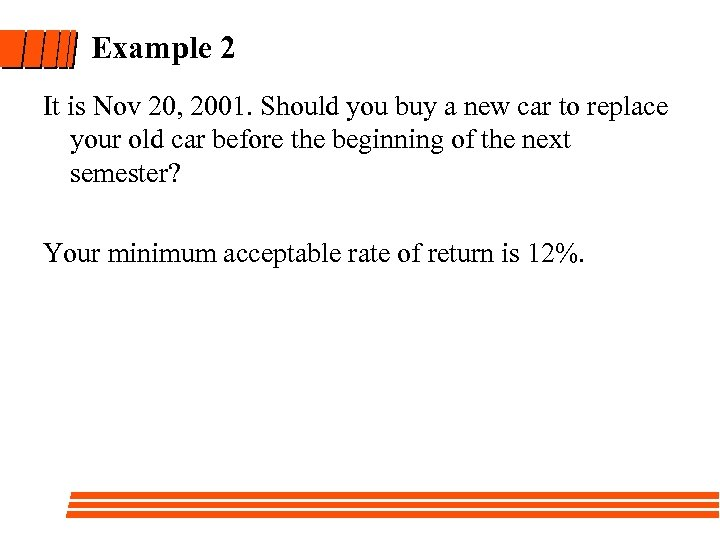Example 2 It is Nov 20, 2001. Should you buy a new car to replace your old car before the beginning of the next semester? Your minimum acceptable rate of return is 12%.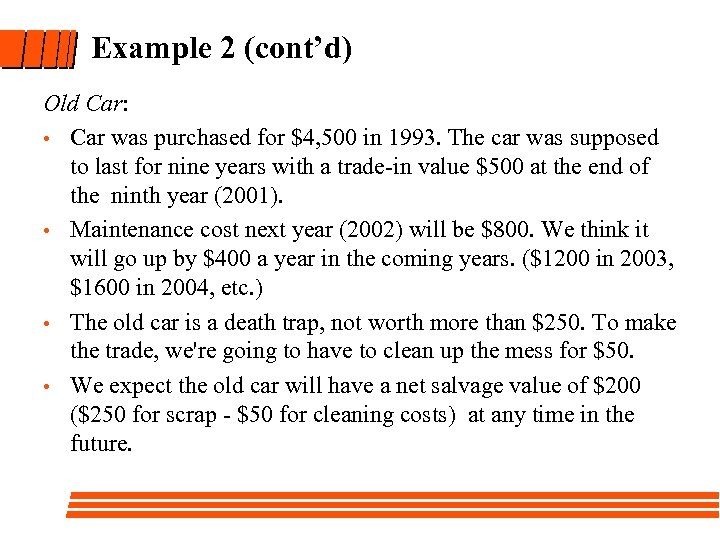Example 2 (cont’d) Old Car: • Car was purchased for \$4, 500 in 1993. The car was supposed to last for nine years with a trade-in value \$500 at the end of the ninth year (2001). • Maintenance cost next year (2002) will be \$800. We think it will go up by \$400 a year in the coming years. (\$1200 in 2003, \$1600 in 2004, etc. ) • The old car is a death trap, not worth more than \$250. To make the trade, we're going to have to clean up the mess for \$50. • We expect the old car will have a net salvage value of \$200 (\$250 for scrap - \$50 for cleaning costs) at any time in the future.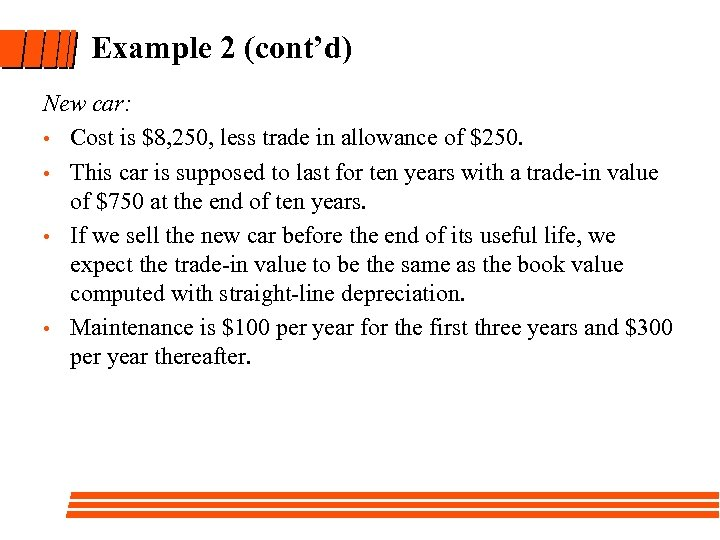Example 2 (cont’d) New car: • Cost is \$8, 250, less trade in allowance of \$250. • This car is supposed to last for ten years with a trade-in value of \$750 at the end of ten years. • If we sell the new car before the end of its useful life, we expect the trade-in value to be the same as the book value computed with straight-line depreciation. • Maintenance is \$100 per year for the first three years and \$300 per year thereafter.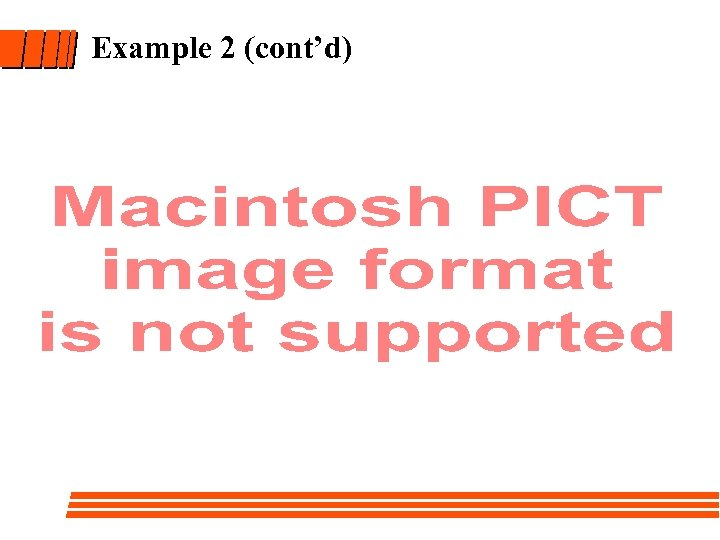Example 2 (cont’d)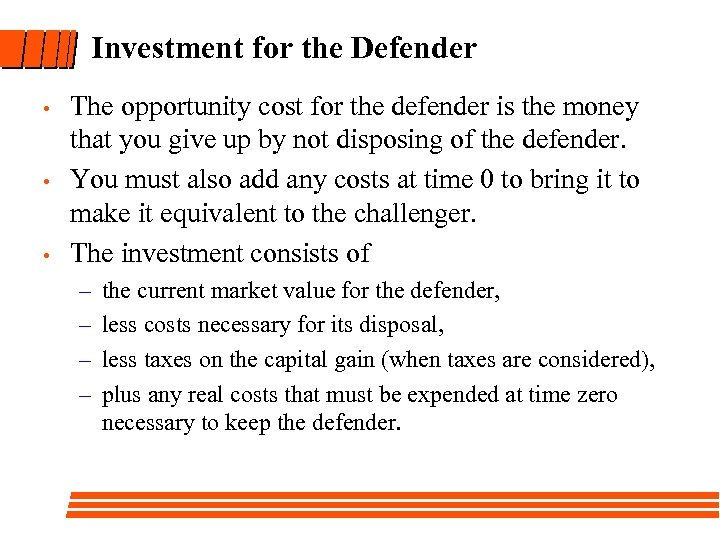Investment for the Defender • • • The opportunity cost for the defender is the money that you give up by not disposing of the defender. You must also add any costs at time 0 to bring it to make it equivalent to the challenger. The investment consists of – – the current market value for the defender, less costs necessary for its disposal, less taxes on the capital gain (when taxes are considered), plus any real costs that must be expended at time zero necessary to keep the defender.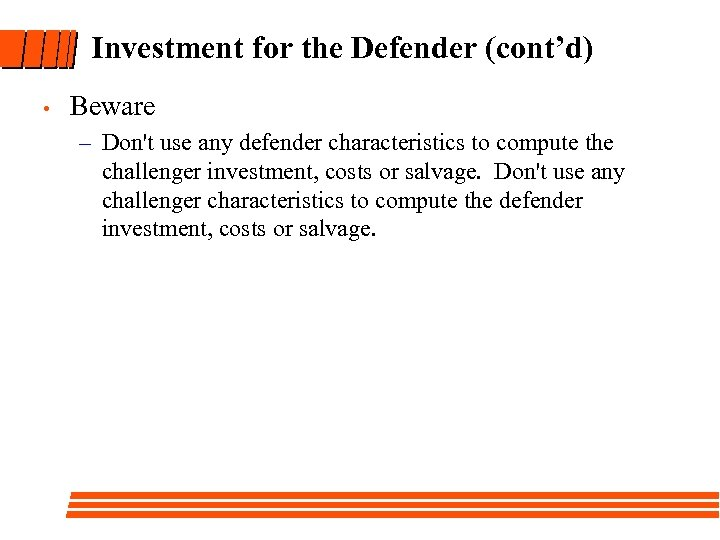Investment for the Defender (cont’d) • Beware – Don't use any defender characteristics to compute the challenger investment, costs or salvage. Don't use any challenger characteristics to compute the defender investment, costs or salvage.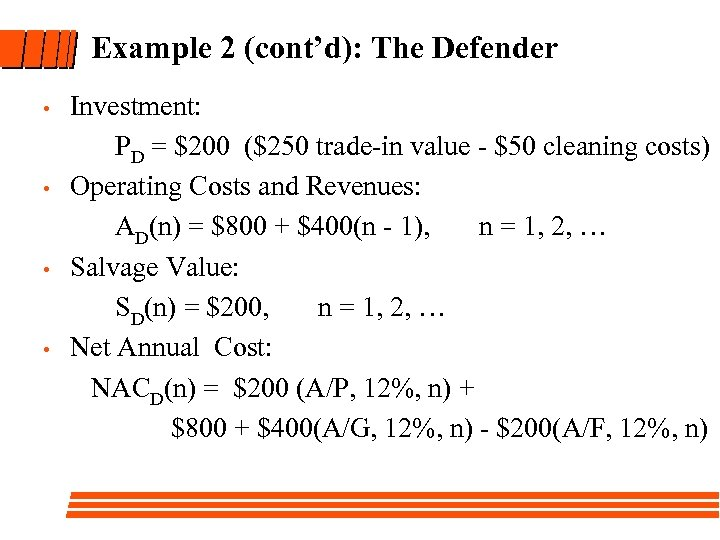Example 2 (cont’d): The Defender • • Investment: PD = \$200 (\$250 trade-in value - \$50 cleaning costs) Operating Costs and Revenues: AD(n) = \$800 + \$400(n - 1), n = 1, 2, … Salvage Value: SD(n) = \$200, n = 1, 2, … Net Annual Cost: NACD(n) = \$200 (A/P, 12%, n) + \$800 + \$400(A/G, 12%, n) - \$200(A/F, 12%, n)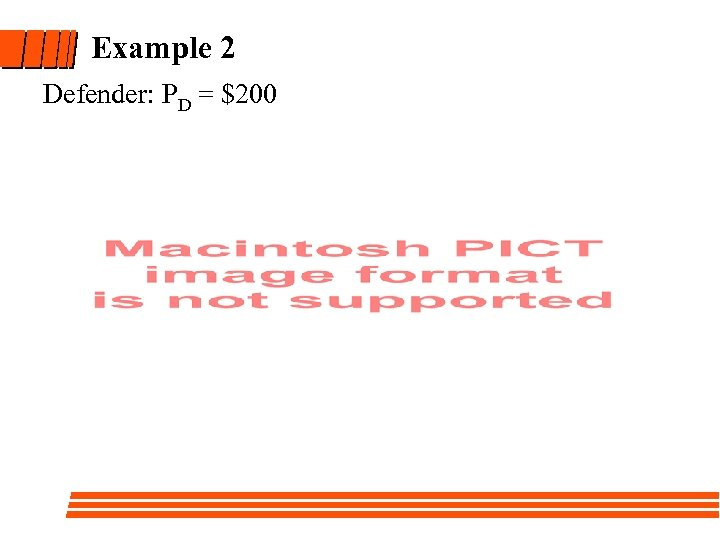Example 2 Defender: PD = \$200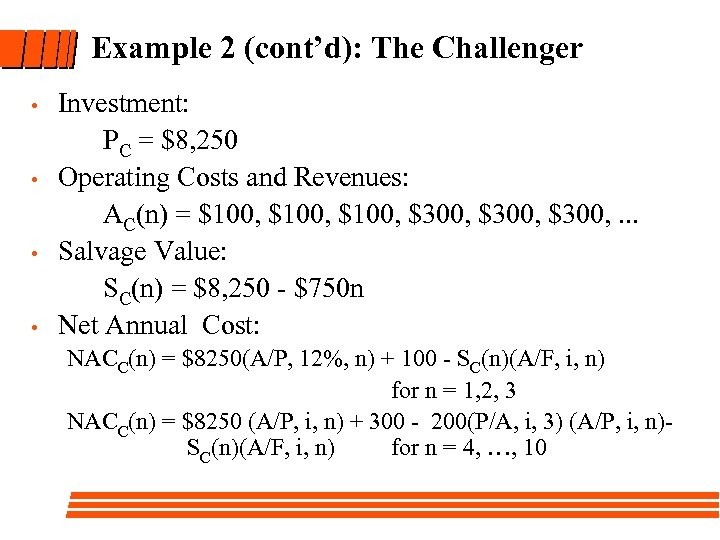Example 2 (cont’d): The Challenger • • Investment: PC = \$8, 250 Operating Costs and Revenues: AC(n) = \$100, \$300, . . . Salvage Value: SC(n) = \$8, 250 - \$750 n Net Annual Cost: NACC(n) = \$8250(A/P, 12%, n) + 100 - SC(n)(A/F, i, n) for n = 1, 2, 3 NACC(n) = \$8250 (A/P, i, n) + 300 - 200(P/A, i, 3) (A/P, i, n)- SC(n)(A/F, i, n) for n = 4, …, 10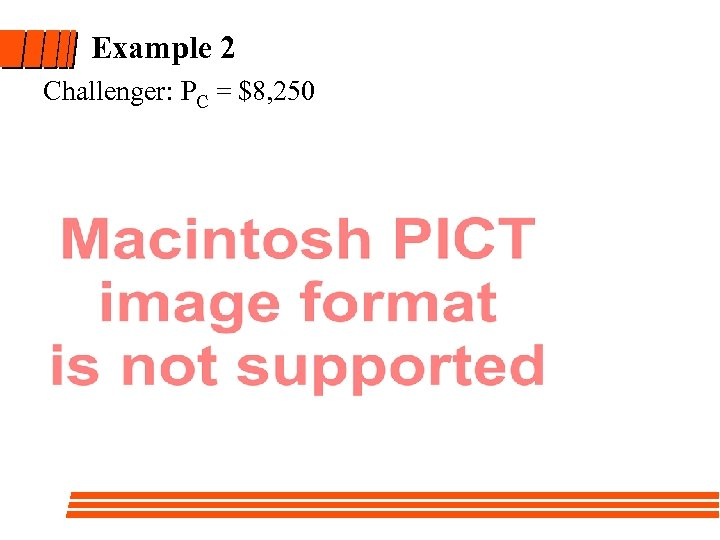Example 2 Challenger: PC = \$8, 250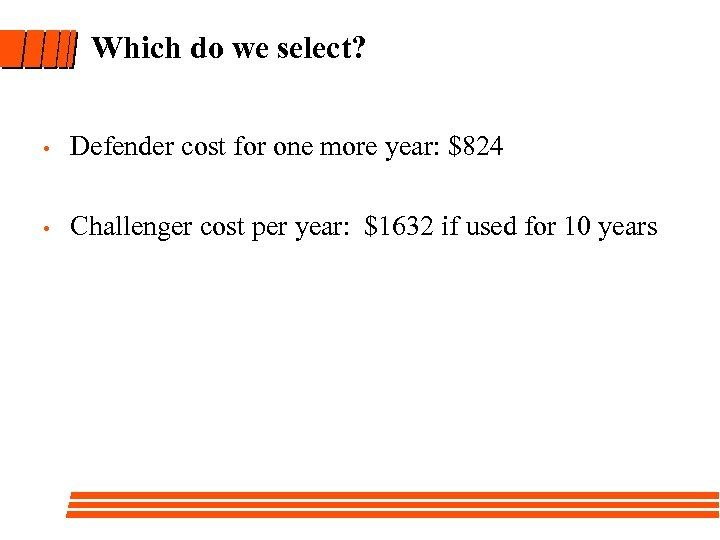Which do we select? • Defender cost for one more year: \$824 • Challenger cost per year: \$1632 if used for 10 years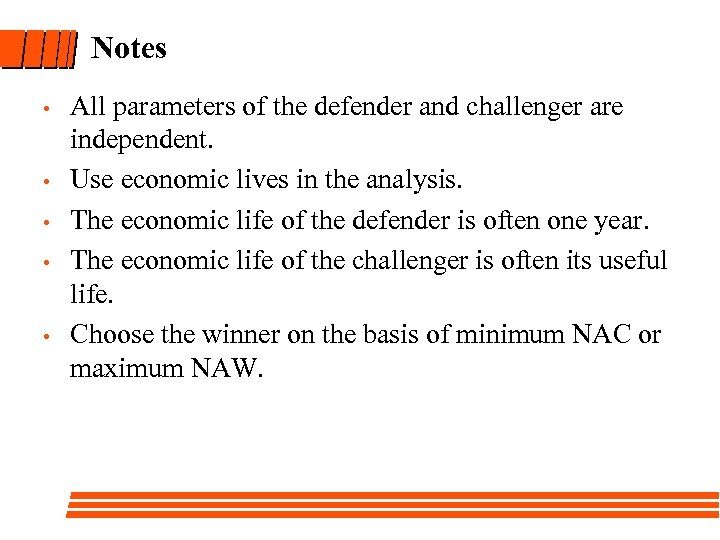Notes • • • All parameters of the defender and challenger are independent. Use economic lives in the analysis. The economic life of the defender is often one year. The economic life of the challenger is often its useful life. Choose the winner on the basis of minimum NAC or maximum NAW.